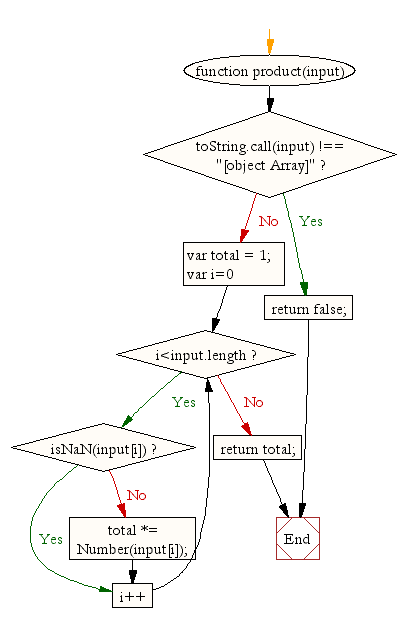# JavaScript: Calculate the product of values in an array

## JavaScript Math: Exercise-18 with Solution

Write a JavaScript function to calculate the product of values in an array.

Test Data:
console.log(product([1,2,3]));
console.log(product([100,-200,3]));
console.log(product([1,2,'a',3]));
Output:
6
-60000
6

Pictorial Presentation:Sample Solution:-

HTML Code:

``````<!DOCTYPE html>
<html>
<meta charset="utf-8">
<title>Product of values in an array</title>
<body></body>
</html>
```
```

JavaScript Code:

``````function product(input){
if (toString.call(input) !== "[object Array]")
return false;

var total   =   1;

for(var i=0;i<input.length;i++){
if(isNaN(input[i])){
continue;
}
total   *=  Number(input[i]);
}
}
console.log(product([1,2,3]));
console.log(product([100,-200,3]));
console.log(product([1,2,'a',3]));
```
```

Sample Output:

```6
-60000
6
```

Flowchart:Live Demo:

See the Pen javascript-math-exercise-18 by w3resource (@w3resource) on CodePen.

Improve this sample solution and post your code through Disqus

What is the difficulty level of this exercise?

Test your Programming skills with w3resource's quiz.

﻿